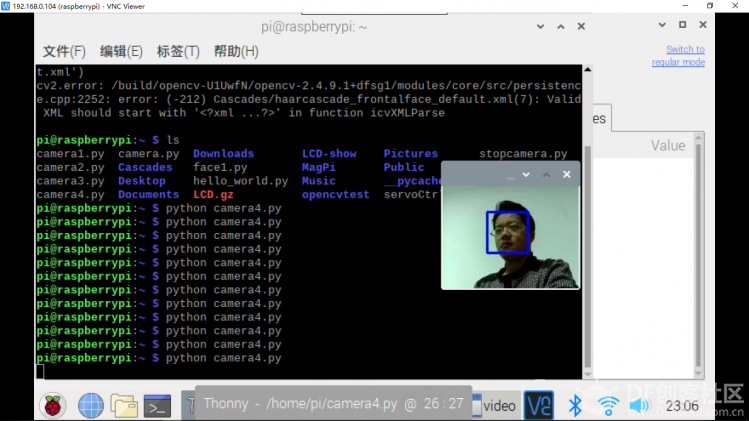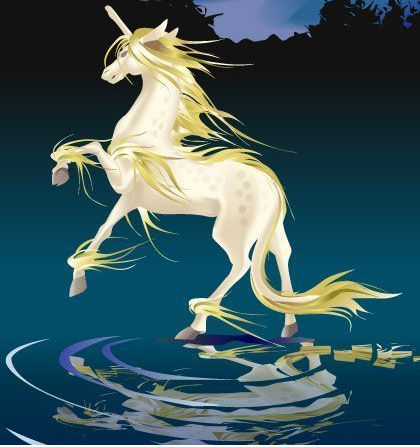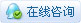# [讨论交流] 【树莓派】人脸追踪（2）树莓派+OpenCV

最常见的人脸检测方式是使用「Haar 级联分类器」。使用基于 Haar 特征的级联分类器的目标检测是 Paul Viola 和 Michael Jones 2001 年在论文《Rapid Object Detection using a Boosted Cascade of Simple Features》中提出的一种高效目标检测方法。这种机器学习方法基于大量正面、负面图像训练级联函数，然后用于检测其他图像中的对象。这里，我们将用它进行人脸识别。最初，该算法需要大量正类图像（人脸图像）和负类图像（不带人脸的图像）来训练分类器。然后我们需要从中提取特征。好消息是 OpenCV 具备训练器和检测器。如果你想要训练自己的对象分类器，如汽车、飞机等，你可以使用 OpenCV 创建一个。
如果不想创建自己的分类器，OpenCV 也包含很多预训练分类器，可用于人脸、眼睛、笑容等的检测。相关的 XML 文件可从该目录下载：[Python] 纯文本查看 复制代码
```import numpy as np
import cv2

cap = cv2.VideoCapture(0)
cap.set(3,640) # set Width
cap.set(4,480) # set Height

while True:
#img = cv2.flip(img, -1)
gray = cv2.cvtColor(img, cv2.COLOR_BGR2GRAY)
gray,
scaleFactor=1.2,
minNeighbors=5,
minSize=(20, 20)
)

for (x,y,w,h) in faces:
cv2.rectangle(img,(x,y),(x+w,y+h),(255,0,0),2)
roi_gray = gray[y:y+h, x:x+w]
roi_color = img[y:y+h, x:x+w]

cv2.imshow('video',img)

k = cv2.waitKey(30) & 0xff
if k == 27: # press 'ESC' to quit
break

cap.release()
cv2.destroyAllWindows()```

cap.set(3,160) # set Width
cap.set(4,120) # set Height

 faces = faceCascade.detectMultiScale(         gray,              scaleFactor=1.2,         minNeighbors=5,              minSize=(20, 20)     )

gray 表示输入 grayscale 图像。
scaleFactor 表示每个图像缩减的比例大小。
minNeighbors 表示每个备选矩形框具备的邻近数量。数字越大，假正类越少。
minSize 表示人脸识别的最小矩形大小。

 for (x,y,w,h) in faces:     cv2.rectangle(img,(x,y),(x+w,y+h),(255,0,0),2)     roi_gray = gray[y:y+h, x:x+w]     roi_color = img[y:y+h, x:x+w]

img = cv2.flip(img, -1)

import numpy as np
import cv2

cv2.imshow("original",img)

#水平镜像
h_flip=cv2.flip(img,1)
cv2.imshow("Flipped Horizontally",h_flip)

#垂直镜像
v_flip=cv2.flip(img,0)
cv2.imshow("Flipped Vertically",v_flip)

#水平垂直镜像
hv_flip=cv2.flip(img,-1)
cv2.imshow("Flipped Horizontally & Vertically",hv_flip)

#平移矩阵[[1,0,-100],[0,1,-12]]
M=np.array([[1,0,-100],[0,1,-12]],dtype=np.float32)
img_change=cv2.warpAffine(img,M,(300,300))
cv2.imshow("translation",img_change)

#90度旋转
rows,cols=img.shape[:2]
M=cv2.getRotationMatrix2D((cols/2,rows/2),90,1)
dst=cv2.warpAffine(img,M,(cols,rows))
cv2.imshow("90",dst)

#45度旋转
rows,cols=img.shape[:2]
M=cv2.getRotationMatrix2D((cols/2,rows/2),45,1)
dst=cv2.warpAffine(img,M,(cols,rows))
cv2.imshow("45",dst)

#缩放
height,width=img.shape[:2]
res=cv2.resize(img,(2*width,2*height))
cv2.imshow("large",res)

# 仿射变换
#对图像进行变换（三点得到一个变换矩阵）
# 我们知道三点确定一个平面，我们也可以通过确定三个点的关系来得到转换矩阵
# 然后再通过warpAffine来进行变换
point1=np.float32([[50,50],[300,50],[50,200]])
point2=np.float32([[10,100],[300,50],[100,250]])

M=cv2.getAffineTransform(point1,point2)
dst1=cv2.warpAffine(img,M,(cols,rows),borderValue=(255,255,255))
cv2.imshow("affine transformation",dst1)
cv2.waitKey(0)

http://shumeipai.nxez.com/2018/03/09/real-time-face-recognition-an-end-to-end-project-with-raspberry-pi.html### kylinpoet中级技匠

 多谢分享，学习了。有料

 您需要登录后才可以回帖 登录 | 立即注册 本版积分规则 回帖并转播 回帖后跳转到最后一页

[[wsData.name]]

#### 硬件清单

• [[d.name]]#### 楼主的其它帖子